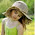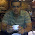## Monday, August 6, 2012

### awk - Passing awk variables to shell

In one of our earlier articles, we discussed how to access or pass shell variables to awk. In this, we will see how to access the awk variables in shell? Or How to access awk variables as shell variables ?  Let us see the different ways in which we can achieve this.

Let us consider a file with the sample contents as below:
```\$ cat file
Linux 20
Solaris 30
HPUX 40
```
1. Access the value of the entry "Solaris" in a shell variable, say x:
```\$ x=`awk '/Solaris/{a=\$2;print a}' file`
\$ echo \$x
30
```
This approach is fine as long as we want to access only one value. What if we have to access multiple values in shell?

2. Access the value of "Solaris" in x, and "Linux" in y:
`\$ z=`awk '{if(\$1=="Solaris")print "x="\$2;if(\$1=="Linux")print "y="\$2}' file``
```\$ echo "\$z"
y=20
x=30```
`\$ eval \$z`
```\$ echo \$x
30
\$ echo \$y
20
```
awk sets the value of "x" and "y" awk variables and prints which is collected in the shell variable "z". The eval command evaluates the variable meaning it executes the commands present in the variable. As a result, "x=30" and "y=20" gets executed, and they become shell variables x and y with appropriate values.

3. Same using the sourcing method:
`\$ awk '{if(\$1=="Solaris")print "x="\$2;if(\$1=="Linux")print "y="\$2}' file > f1`
`\$ source f1`
```\$ echo \$x
30
\$ echo \$y
20
```
Here, instead of collecting the output of awk command in a variable, it is re-directed to a temporary file. The file is then sourced or in other words executed in the same shell. As a result, "x" and "y" become shell variables.
Note: Depending on the shell being used, the appropriate way of sourcing has to be done. The "source" command is used here since the default shell is bash.1.1.